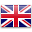Live ChatEnglish
Home / News / Understanding Resistors: Everything You Need to Know

# Understanding Resistors: Everything You Need to Know

As an electronics enthusiast, I know that resistors are an essential component in electronic circuits. They play a crucial role in controlling the flow of electric current and voltage in a circuit, and without them, electronic devices would not function correctly. In this article, I will explain everything you need to know about resistors, including their types, how they work, how to calculate resistance, and much more.

## Introduction to Resistors

A resistor is an electronic component that resists the flow of electric current in a circuit. It is used to control the amount of current and voltage that passes through it. Resistors are essential components in electronic circuits, as they help to regulate the flow of electricity and protect other components from damage caused by excessive current or voltage.

## What are Resistors and How Do They Work?

Resistors are passive electronic components that are designed to limit the flow of electric current in a circuit. They consist of a material that resists the flow of electric current, such as carbon, metal, or a semiconductor material. The resistance of a resistor is measured in ohms, and it is determined by the material it is made of, its size, and its shape.

When electric current flows through a resistor, it encounters resistance, which causes a drop in voltage across the resistor. The amount of resistance offered by a resistor is determined by the ratio of the voltage across the resistor to the current that flows through it, as defined by Ohm’s law. This makes resistors useful in controlling the amount of current and voltage in a circuit.

## Types of Resistors

There are several types of resistors, and each type is designed for a specific application. The most common types of resistors include:

1. Carbon Composition Resistors: These are the oldest and most basic type of resistor. They are made of a mixture of carbon and a binder material, which is then molded into a cylindrical shape. Carbon composition resistors are inexpensive and are used in low-power applications.
2. Film Resistors: These are made by depositing a resistive material on a ceramic substrate. They are available in different types, including metal film, carbon film, and metal oxide film resistors. They are used in high-precision applications.
3. Wirewound Resistors: These are made by winding a resistive wire around a ceramic or fiberglass core. They are used in high-power applications.
4. Variable Resistors: These are resistors whose resistance can be adjusted manually or electronically. They are used in applications where the resistance needs to be adjusted, such as volume or tone controls.

## Resistor Color Code Chart

Resistors are color-coded to indicate their resistance value, tolerance, and sometimes their wattage rating. The color code chart is a standardized system that is used to indicate the value of the resistance of a resistor. The chart consists of four or five colored bands that are painted on the resistor, and each band represents a digit or a multiplier.

The first two bands represent the significant figures of the resistance value, the third band represents the multiplier, and the fourth band represents the tolerance. Some resistors also have a fifth band that represents the temperature coefficient.

## How to Calculate Resistance

The resistance of a resistor can be calculated using Ohm’s law, which states that the resistance of a conductor is equal to the voltage across the conductor divided by the current flowing through it. The formula for calculating resistance is R = V/I, where R is the resistance, V is the voltage, and I is the current.

## Uses of Resistors in Electronics

Resistors are used in a wide range of electronic applications, including:

1. Voltage Dividers: Resistors are used in voltage divider circuits to reduce the voltage of a signal.
2. Current Limiters: Resistors are used to limit the amount of current flowing through a circuit.
3. Timing Circuits: Resistors are used in timing circuits to control the frequency of a signal.
4. Filters: Resistors are used in filters to remove unwanted frequencies from a signal.
5. Temperature Sensors: Resistors are used as temperature sensors in electronic devices.

## Common Mistakes to Avoid When Working with Resistors

When working with resistors, there are several common mistakes that you should avoid, including:

1. Using the wrong resistor value.
2. Overheating the resistor.
3. Failing to account for the tolerance of the resistor.
4. Failing to account for the power rating of the resistor.
5. Failing to use the correct technique when soldering the resistor.

## Tips for Choosing the Right Resistor

When choosing a resistor, there are several factors to consider, including:

1. The resistance value.
2. The power rating.
3. The tolerance.
4. The temperature coefficient.
5. The type of resistor.

## Testing Resistors with a Multimeter

To test a resistor using a multimeter, you will need to set the multimeter to the resistance measurement mode. Then, connect the multimeter leads to the ends of the resistor and read the resistance value on the multimeter.

### Conclusion – Importance of Resistors in Electronics

In conclusion, resistors are essential components in electronic circuits, and they play a crucial role in controlling the flow of electric current and voltage in a circuit. There are several types of resistors, each designed for a specific application, and they are used in a wide range of electronic devices. Understanding resistors and how to choose and work with them is essential for anyone interested in electronics.